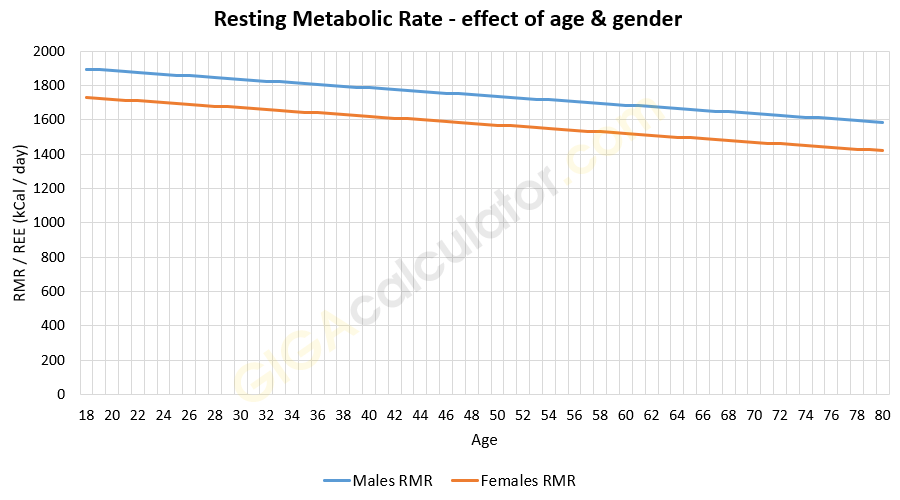# Resting Metabolic Rate Calculator

Use this RMR calculator a.k.a. resting calorie burn calculator to easily calculate your Resting Metabolic Rate (RMR) which is how many kcal per day you need if you are just resting. It is a slightly less strict measurement than BMR. A synonymous term is Resting Energy Expenditure (REE).

years
ft
in

### Calculation results

1,664 Calories per day RMR
Share calculator:

Embed this tool:
get code

RMR metrics used: 1 Calorie = 1 kcal (1 kilocalorie) = 1,000 calories.

## What is RMR?

The Resting Metabolic Rate (RMR) is the amount of energy your body consumes when in resting state. It is also encountered under the names Resting Energy Expenditure or REE, as well as RDEE - Resting Daily Energy Expenditure, which is why this tool can be referred to as both an RMR calculator, REE calculator, or RDEE calculator. Abbreviations aside, RMR is a huge component of Total Daily Energy Expenditure (TDEE) - usually 60-85% of TDEE and is often used to calculate daily caloric needs, and from them: different kinds of diets / food regimes, and nutrient requirements.

This is also the general application of a Resting Metabolic Rate calculator: calculating metabolism needs, fitness regimen energy requirements, athletes food intake for competitive sports, and so on. Supplement intake and dosage may be governed by the resting metabolic rate as well. Many physical activity trackers, including Android & iOS apps would have an in-built calculator.

RMR is very similar to BMR, but is measured under less strict conditions leading to it having slightly higher values than BMR. The difference is usually so small that in medical literature the two terms can be seen used as synonyms. Under laboratory / clinical conditions resting metabolic rate is measured using the volume of oxygen intake a person has in resting condition. Using our online resting metabolic state (RMR) calculator one can estimate a person's RMR with a decent amount of accuracy, based on population averages. Read more on RMR vs BMR in the context of weight loss.

## RMR formula and calculating your Resting Metabolic Rate

The formula we use in this calculator is different for males and females. It takes into account age (years), weight (kg), and height (stature in cm). The equation for males is:

66 + (13.7 x weight) + (5 x height) - (6.8 x age)

And for females it is:

655 + (9.6 x weight) + (1.85 x height) - (4.7 x age)

The formulas are from Katch, McArdle & Katch (2011) . In order to manually calculate your RMR you need to perform the necessary unit conversions, then replace the respective values in the formula for your gender. Our tool does this automatically, as required.

## How accurate are RMR / REE estimations?

Some call into question the established formulas used in many RMR calculations, for example Owen et al.  state that for healthy women there is between 7% and 14% overestimation using the current formulas. However, this conclusion is based on only 44 women aged 18-65 and it is unclear how representative a sample they are for the general population. There were 8 trained athletes among the 44 subjects of the study (18%), most likely way higher than the general population.

More recent and much larger studies like Wright et al.  found RMR estimation to be accurate to ±10% in only around 44% of individual cases when the person was classified as overweight (BMI>25) and obese (BMI>30), especially in the second case. However, their statistical analysis, sadly, gives no indication of the direction of the bias. A much larger study by Marra et al.  estimates the ±10% accuracy of estimation to be round 55% for obese patients (BMI>30) aged 18-65. They, however, also note that using obese-specific REE formulas do not improve prediction rates compared to equations based on normal-weight subjects. According, again, to Marra et al. including body fat percentage or fat free mass percentage does not improve predictions versus those based on weight, height, age, and gender alone.

It should be noted, though, that in the above two papers only one of the equations being examined had a fine age gradient, the rest had coarse boundaries such as 18-29, 30-59, 60+, making them much less accurate than the formula we use in this RMR calculator, which takes age into more consideration, as seen below:The RMR graph is for a male and female of different age but same mass and stature. The effect of gender is also clearly visible with women having lower average resting energy expenditure. The figure can be replicated for any dataset of interest, as long as it can be broken down by men and women.

Still, in all cases where medical needs make it necessary to accurately measure RMR / REE, indirect calorimetry (IC) should be undertaken. IC is a non-invasive procedure, generally considered the gold standard in clinical practice, however the cost and time, as well as the availability of a clinic with the necessary equipment should be considered. Our resting energy expenditure calculator remains an inexpensive and decently accurate alternative for less demanding cases.